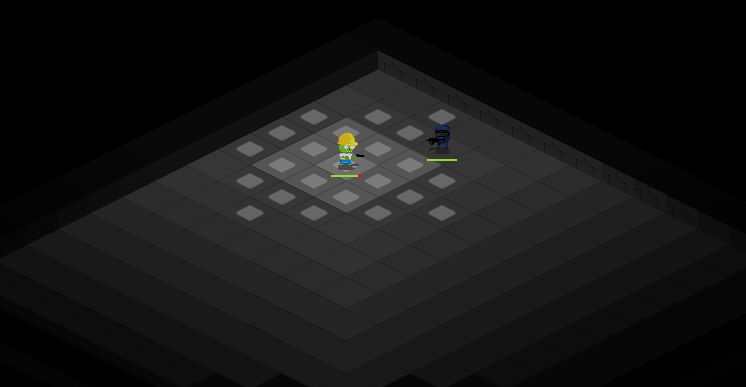# Flood Fill

The flood-fill algorithm determines the area connected to a given node in a multidimensional array. Using connection rules to establish if an adjacent node is connected or blocked you can "fill" all surrounding nodes to any desired depth.

The algorithm takes three parameters: a start node, a target fill function, a target block detection function and optionally a maximum range or depth.## Flood Fill Usage

Flood-fill is the same algorithm used in paint bucket functions of image editors. I have put it to use as a character's available travel cells in 2D games. It is recursive in nature and can be expanded for any number of "adjacent" direction checks, though most applications are for 4 direction space.

In this example we are rendering available movement positions for a player's turn. A flood fill takes the players speed as the range and sweeps all non-blocking structures to display places the player can travel to.

``````void LevelClient::renderMoveMarkers()
{
unsigned int i=0;
LevelClient* lc = this;
bit::FloodFill::compute(playerTile->schema.x / tileWidth, playerTile->schema.y / tileHeight,
// Lambda function to take a cell's coordinate, find it in our map, and create a movement marker over it
[lc, &i] (int x, int y, int depth) {
TileClient* tile = lc->getTileAtIndices(x, y);
// This check was to not duplicate markers because floodfill will inspect the same one more than once
{

sf::Color w(255, 255, 255);
lc->moveMarkers[i].renderAt(x * lc->tileWidth, y * lc->tileHeight, w);
i++;
}
},
// This is our optional block check routine, which looks for the tile being checked and sees if it has an obstacle on it
[lc] (int x, int y, int depth) -> bool {
TileClient* tile = lc->getTileAtIndices(x, y);
return !tile || depth > lc->playerCharacter->schema.speed || (tile->hasBody && tile->bodyClient != lc->playerCharacter);
}
);
}``````

## Implementation in C++

The algorithm was implemented for 4 directional use cases. In addition the multi-dimensional array has been abstracted away and replaced with simple x and y coordinate math and lambda functions to map the coordinates back to implementation data.

`FloodFill.hpp`

``````#pragma once
#ifndef BIT_FLOODFILL_H
#define BIT_FLOODFILL_H

#include <functional>
#include <vector>

namespace bit
{
class FloodFill
{
public:

static unsigned int floodfillId;

static void compute(int x, int y, std::function<void(int, int, int)> inspect, std::function<bool(int, int, int)> isBlocked);

private:
static void compute(int x, int y, std::function<void(int, int, int)> inspect, std::function<bool(int, int, int)> isBlocked, int depth);

};
}
#endif``````

`FloodFill.cpp`

``````#include "FloodFill.hpp"

unsigned int bit::FloodFill::floodfillId = 0;

void bit::FloodFill::compute(int x, int y, std::function<void(int, int, int)> inspect, std::function<bool(int, int, int)> isBlocked)
{
floodfillId++;
compute(x, y, inspect, isBlocked, 0);
}

void bit::FloodFill::compute(int x, int y, std::function<void(int, int, int)> inspect, std::function<bool(int, int, int)> isBlocked, int depth)
{
if(isBlocked(x, y, depth))
return;

inspect(x, y, depth);

depth++;

compute(x + 1, y, inspect, isBlocked, depth);
compute(x - 1, y, inspect, isBlocked, depth);
compute(x, y + 1, inspect, isBlocked, depth);
compute(x, y - 1, inspect, isBlocked, depth);
}``````